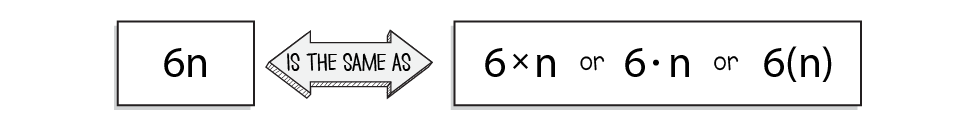# Lesson 2: Multiplication Review

## Getting Started

In this lesson, you'll review how to multiply both whole and decimal numbers. First, review some basic facts about multiplication:
• Multiplication is repeated addition. In other words, 4 × 3 means "4 groups of 3" and is the same as 3 + 3 + 3 + 3.
• The numbers being multiplied are called factors, and the answer to a multiplication problem is the product.
• Multiplication can be represented in the following ways:You are already familiar with the multiplication symbol (×), but you can also represent multiplication using something called the dot operator (∙). That means you can show "five times two" as "5 × 2" or as "5 ∙ 2". The dot operator is useful for problems involving variables, which are letters used to represent an unknown number. (You'll learn more about variables in Unit 4.) For example, "5 × x" is a little confusing since the variable "x" and the multiplication symbol look very similar. In this case, "5 ∙ x" is much clearer.

You can also use parentheses to represent multiplication. When two numbers are being multiplied, you can write one inside the parentheses: 5(9). The two numbers are written right next to each other, and no other symbol besides the parentheses is given. The parentheses indicate that the 5 and the 9 should be multiplied.

There is one more way multiplication is represented, and it is used with problems involving those variables just mentioned. If you have a number and a variable, or two or more variables, you can write them right next to each other to show multiplication.It's important to note that this form can only be used if there is a variable letter involved. We don't use this form with numbers because if we did, we wouldn't know if 35 meant "thirty-five" or "three times five." How confusing would that be!

### Stuff You Need

• 3-hole drilled card stock (kit)
• colored pencils
• construction paper (kit)
• glue or glue stick
• Interactive Notebook
• marker
• scissors

• How is multiplication like repeated addition?
• How does the use of place value to write numbers make it easy to multiply or divide by powers of 10?

### Things to Know

• Multiplication is repeated addition. For example, 4 × 3 means "4 groups of 3" and is the same as 3 + 3 + 3 + 3.
• The numbers being multiplied are called factors, and the answer to a multiplication problem is called the product.
• You can show multiplication using the multiplication symbol (×), the dot operator (∙), or parentheses.
• A property of something is a characteristic that applies to all instances of that thing.
• The commutative property of multiplication states that you can multiply numbers in any order. For example, 3 × 5 has the same answer as 5 × 3.
• The associative property of multiplication says that it doesn't matter how the factors are grouped in a multiplication problem. For example, the problem
(12 × 2) × 5 can be regrouped as 12 × (2 × 5).
• The distributive property of multiplication states that multiplying the sum of two numbers by a factor is the same as multiplying both numbers by the factor and then adding the products.

### Skills

• Compute fluently with multi-digit numbers
• Apply properties of operations as strategies to multiply rational numbers
• Fluently multiply multi-digit decimals using the standard algorithm
• Solve real-world and mathematical problems involving the four operations with rational numbers

### Introducing the Lesson

In this lesson your child will review and practice multiplying multi-digit whole and decimal numbers. She should already have mastered her multiplication fact (times tables) through 12. If she would benefit from reviewing these facts, she can use the multiplication practice tool at the following web link: